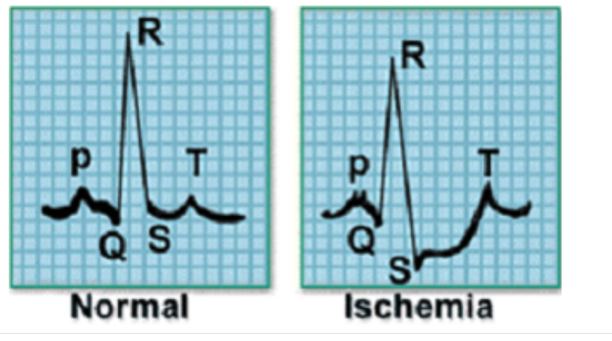# Run this cell to install the latest version of fastai2 shared on github
!pip install git+https://github.com/fastai/fastai2.git

# Run this cell to install the latest version of fastcore shared on github
!pip install git+https://github.com/fastai/fastcore.git

# Run this cell to install the latest version of timeseries shared on github
!pip install git+https://github.com/ai-fast-track/timeseries.git

%reload_ext autoreload
%matplotlib inline

from fastai2.basics import *

from timeseries.all import *


# Class Activation Map (CAM) and Grafient-CAM (GRAD-CAM) Tutorial

Both CAM and GRAD-CAM allow producing ‘visual explanations’ on how a Convolutional Neural Network (CNN) model based its classification and therefore help interpreting the obtained results. The InceptionTime model is used as an illustration in this notebook.

## ECG Dataset

This dataset was formatted by R. Olszewski as part of his thesis “Generalized feature extraction for structural pattern recognition in time-series data,” at Carnegie Mellon University, 2001. Each series traces the electrical activity recorded during one heartbeat. The two classes are a normal heartbeat and a Myocardial Infarction. Cardiac ischemia refers to lack of blood flow and oxygen to the heart muscle. If ischemia is severe or lasts too long, it can cause a heart attack (myocardial infarction) and can lead to heart tissue death.## Training a model

# You can choose any of univariate dataset listed the data.py file
path = unzip_data(URLs_TS.UNI_ECG200)

dsname =  'ECG200' # 'GunPoint'
fname_train = f'{dsname}_TRAIN.arff'
fname_test = f'{dsname}_TEST.arff'
fnames = [path/fname_train, path/fname_test]
fnames

[Path('/home/farid/.fastai/data/ECG200/ECG200_TRAIN.arff'),
Path('/home/farid/.fastai/data/ECG200/ECG200_TEST.arff')]
# num_workers=0 is for Windows platform

dls.show_batch(chs=range(0,12,3))

learn = ts_learner(dls)
learn.fit_one_cycle(25, lr_max=1e-3)

epoch train_loss valid_loss accuracy time
0 1.477478 0.692645 0.575000 00:01
1 1.418432 0.693228 0.300000 00:01
2 1.247476 0.694551 0.425000 00:01
3 1.169967 0.695732 0.425000 00:01
4 1.026473 0.697049 0.425000 00:01
5 0.921037 0.700040 0.425000 00:01
6 0.831905 0.702114 0.425000 00:01
7 0.760788 0.703392 0.425000 00:01
8 0.701200 0.708715 0.425000 00:01
9 0.652415 0.708759 0.425000 00:01
10 0.610008 0.705038 0.425000 00:01
11 0.573161 0.698569 0.425000 00:01
12 0.541027 0.683905 0.425000 00:01
13 0.512720 0.661773 0.500000 00:01
14 0.487877 0.634480 0.575000 00:01
15 0.466010 0.602281 0.750000 00:01
16 0.446488 0.561948 0.850000 00:01
17 0.428792 0.512738 0.950000 00:01
18 0.413006 0.466702 0.975000 00:01
19 0.398709 0.428802 0.950000 00:01
20 0.385927 0.400146 0.925000 00:01
21 0.374558 0.374902 0.925000 00:01
22 0.364030 0.356121 0.925000 00:01
23 0.354384 0.340358 0.925000 00:01
24 0.345539 0.328972 0.925000 00:01
learn.show_results()

model = learn.model.eval()

model

SequentialEx(
(layers): ModuleList(
(0): InceptionModule(
(bottleneck): Conv1d(128, 32, kernel_size=(1,), stride=(1,))
(convs): ModuleList(
(0): Conv1d(32, 32, kernel_size=(39,), stride=(1,), padding=(19,), bias=False)
(1): Conv1d(32, 32, kernel_size=(19,), stride=(1,), padding=(9,), bias=False)
(2): Conv1d(32, 32, kernel_size=(9,), stride=(1,), padding=(4,), bias=False)
)
(maxpool_bottleneck): Sequential(
(0): MaxPool1d(kernel_size=3, stride=1, padding=1, dilation=1, ceil_mode=False)
(1): Conv1d(128, 32, kernel_size=(1,), stride=(1,), bias=False)
)
(bn_relu): Sequential(
(0): BatchNorm1d(128, eps=1e-05, momentum=0.1, affine=True, track_running_stats=True)
(1): ReLU()
)
)
(1): Shortcut(
(act_fn): ReLU(inplace=True)
(conv): Conv1d(128, 128, kernel_size=(1,), stride=(1,), bias=False)
(bn): BatchNorm1d(128, eps=1e-05, momentum=0.1, affine=True, track_running_stats=True)
)
)
)
dls.vocab

(#2) ['-1','1']

## Decoding class labels

# i2o() function
# Converting CategoryTensor label into the human-readable label
lbl_dict = dict([
(0, 'Normal'),
(1, 'Myocardial Infarction')]
)
def i2o(y):
return lbl_dict.__getitem__(y.data.item())
# return lbl_dict.__getitem__(int(dls.tfms.decodes(y)))


## Creating a customized batch : list of 2 items

- batch: corresponds to Normal ECG

• batch: corresponds to Myocardial Infarction
idxs = [0,2]
batch = get_batch(dls.train.dataset, idxs)


## Plotting CAM for several dataset items in one shared figure

Example:the function expects a list of items and plots CAM for the provided items list.

### Class Activation Map (CAM)

This option calculates the activations values at the selected layer.By default the activations curves are plotted in one single figure.

func_cam=cam_acts : activation function name (activation values at the chosen model layer). It is the default value

The figure title [Myocardial Infarction - Normal] - CAM - mean should be read as follow:

• Myocardial Infarction : class of the first curve
• Normal : class of the second curve
• CAM : activation function name (activation values at the chosen model layer)
• mean : type of reduction (read the explanation below: 4 types of reductions)
show_cam(batch, model, layer=5, i2o=i2o, func_cam=cam_acts) # default:  func_cam=cam_acts, multi_fig=False, figsize=(6,4)


### Using CAM and CMAP.seismic palette

show_cam(batch, model, layer=5, i2o=i2o, multi_fig=True, cmap=CMAP.seismic) # default: func_cam=cam_acts, figsize=(13,4)


### Using GRAD-CAM and CMAP.seismic palette

Notice the difference in activations values between CAM and GRAD-CAM

show_cam(batch, model, layer=5, i2o=i2o, func_cam=grad_cam_acts, multi_fig=True, cmap=CMAP.seismic)


### Superimposed curves give another insight in comparing the 2 time series

show_cam(batch, model, layer=5, i2o=i2o, func_cam=grad_cam_acts, cmap=CMAP.seismic)


### Plotting scattered lines

show_cam(batch, model, layer=5, i2o=i2o, linewidth=2, scatter=True, cmap=CMAP.hot_r)


## Plotting CAM for a batch of items

We can also feed the show_cam() a full batch return straight from DataLoaders one_batch() method as shown here below

### Creating a batch of 5 items

dls.train = dls.train.new(bs=5)
batch = dls.train.one_batch()
# batch

show_cam(batch, model, layer=5, i2o=i2o, cmap=CMAP.viridis)

show_cam(batch, model, layer=5, i2o=i2o, cmap=CMAP.viridis, multi_fig=True, figsize=(18, 8), linestyles='dotted')

show_cam(batch, model, layer=5, i2o=i2o, func_cam=grad_cam_acts, multi_fig=True, figsize=(18, 8))


## Plotting CAM for a single dataset item

We can also feed the show_cam() a single item

There are also 164 different palettes. Check out CMAP class and its autocompletion

line styles :'solid' | 'dashed' | 'dashdot' | 'dotted'

idxs = 
batch = get_batch(dls.train.dataset, idxs)

show_cam(batch, model, layer=5, i2o=i2o, cmap='rainbow', linewidth=2, linestyles='dotted')
`